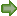STRUCTURAL SCIENCECRYSTAL ENGINEERINGMATERIALS
ISSN: 2052-5206

## Lattice constants and thermal expansion of H2O and D2O Ice Ih between 10 and 265 K. Addendum

aInstitut für Kristallographie, Universität Tübingen, D-72070 Tübingen, Germany, bHasylab/ DESY, Notkestrasse 85, D-22603 Hamburg, Germany, and cGZG Abt. Kristallographie, Universität Göttingen, Goldschmidtstrasse 1, D-37077 Göttingen, Germany
*Correspondence e-mail: wkuhs1@gwdg.de

(Received 30 October 2011; accepted 7 November 2011; online 6 January 2012)

In a previous paper we reported the lattice constants and thermal expansion of normal and deuterated ice Ih [Röttger et al. (1994). Acta Cryst. B50, 644–648]. Synchrotron X-ray powder diffraction data were used to obtain the lattice constants and unit-cell volumes of H2O and D2O ice Ih in the temperature range 15–265 K. A polynomial expression was given for the unit-cell volumes. It turns out that the coefficients quoted have an insufficient number of digits to faithfully reproduce the volume cell data. Here we provide a table with more significant digits. Moreover, we also provide the coefficients of a polynomial fit to the previously published a and c lattice constants of normal and deuterated ice Ih for the same temperature range.

In a previous paper we reported the lattice constants and thermal expansion of normal and deuterated ice Ih (Röttger et al., 1994). Synchrotron X-ray powder diffraction data were used to obtain the lattice constants and unit-cell volumes of H2O and D2O ice Ih in the temperature range 15–265 K. A polynomial expression was given for the unit-cell volumes. It turns out that the coefficients quoted have an insufficient number of digits to faithfully reproduce the volume cell data. This is due to the large correlations amongst the terms of even as well as uneven order. Here we provide a table with more significant digits and also correct one rounding error for the A3 term of the H2O unit-cell volume. Moreover, we also provide the coefficients of a polynomial fit to the previously published a and c lattice constants of normal and deuterated ice Ih for the same temperature range. In Table 1these coefficients are given together with the quality of the fit. The database is identical to that given in Röttger et al. (1994). The coefficients A1 and A2 were set to zero as the thermal expansivity and its temperature derivative are assumed to be 0 at T = 0 K. Under this assumption the validity of the polynomial expressions is from 0 to 265 K. Finally, we like to recall that a many-term polynomial expression was adopted to faithfully represent the measured data and not because there is a particular meaning in the various higher-order terms. We indicate that other approaches could be chosen based on various empirical quasi-harmonic approximations (Reeber & Wang, 1996; Wang & Reeber, 1995) with a different set of parameters; the validity of these approximations for the ice Ih case remains, however, to be proven and will not be attempted here. In concluding we note that the marked isotopic difference of lattice constants between deuterated and normal ice Ih so clearly established by our data is still far from being understood (Herrero & Ramírez, 2011).

 Table 1Coefficients of the polynomial expression Vunit cell(T) = A0 + A1T + A2T2 + A3T3… + A8T8 and a(T), c(T) = A0 + A1T + A2T2 + A3T3… + A8T8
 Uncertainties corresponding to the 90% confidence level are given in brackets and refer to the last digits of the coefficients (ignoring any additional digits given as subscripts in the case of A0). The quality of fit is given as χ2.
Unit-cell volume H2O ice Ih Lattice constant a H2O ice Ih Lattice constant c H2O ice Ih Unit cell volume D2O ice Ih Lattice constant a D2O ice Ih Lattice constant c D2O ice Ih
A0 128.2147 (159) 4.496915 (2) 7.321125 (3) 128.3316 (151) 4.49828 (2) 7.32336 (6)
A1 = A2 0 0 0 0 0 0
A3 −1.3152 (2643) × 10−6 −1.9790 (3205) × 10−8 −2.4944 (6146) × 10−8 −2.2616 (6547) × 10−6 −3.9099 (7361) × 10−8 −3.0567 (1.4264) × 10−8
A4 2.4837 (5228) × 10−8 3.8958 (6356) × 10−10 4.6735 (1.2806) × 10−10 5.1581 (1.7731) × 10−8 9.7883 (2.1911) × 10−10 5.9033 (2.9651) × 10−10
A5 −1.6064 (3876) × 10−10 −2.6930 (4820) × 10−12 −2.9799 (9966) × 10−12 −4.5811 (1.9116) × 10−10 −9.7393 (2.5879) × 10−12 −3.9203 (2.3100) × 10−12
A6 4.6097 (1.2625) × 10−13 8.2861 (1.6256) × 10−15 8.3902 (3.4003) × 10−15 2.0890 (1.0144) × 10−12 4.9329 (1.4896) × 10−14 1.1541 (7936) × 10−14
A7 −4.9661 (1.5196) × 10−16 −9.5759 (2.0415) × 10−18 −8.8400 (4.2799) × 10−18 −4.8591 (2.6388) × 10−15 −1.2573 (4168) × 10−16 −1.2751 (1.0119) × 10−17
A8 0 0 0 4.5747 (2.6900) × 10−1 1.2798 (4546) × 10−19 0
χ2 6.91 8.84 9.36 6.12 10.28 3.97

### ReferencesSTRUCTURAL SCIENCECRYSTAL ENGINEERINGMATERIALS